Courses

# Test: Communication Systems - 6

## 25 Questions MCQ Test Communication Theory | Test: Communication Systems - 6

Description
This mock test of Test: Communication Systems - 6 for Electronics and Communication Engineering (ECE) helps you for every Electronics and Communication Engineering (ECE) entrance exam. This contains 25 Multiple Choice Questions for Electronics and Communication Engineering (ECE) Test: Communication Systems - 6 (mcq) to study with solutions a complete question bank. The solved questions answers in this Test: Communication Systems - 6 quiz give you a good mix of easy questions and tough questions. Electronics and Communication Engineering (ECE) students definitely take this Test: Communication Systems - 6 exercise for a better result in the exam. You can find other Test: Communication Systems - 6 extra questions, long questions & short questions for Electronics and Communication Engineering (ECE) on EduRev as well by searching above.
QUESTION: 1

### A Discone antenna is

Solution:

It is a combination of disc and cone in close approximity.

QUESTION: 2

### In a two tone AM system the two modulating frequencies are 2000 p and 4000 p rad/sec. If carrier frequency is 2p x 106 rad/sec the frequencies of upper sidebands are

Solution: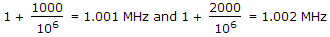.

QUESTION: 3

### For a system to be designated as hi-fi, the signal to noise ratio should be at least

Solution:

Hi-Fi system has a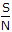ratio at least equal to 50 dB.

QUESTION: 4

Dolby system of noise reduction divides the total audio spectrum into

Solution:

Dolby A system divides the frequency in 4 bands viz.f < 80 Hz, 80 Hz < f < 2999 Hz, f > 3000 Hz, f > 9000 Hz.

QUESTION: 5

In a system an input resistance of 20 kΩ causes a noise voltage of 15μV. If two such input resistances each of 20 kΩ are used in parallel, the total noise voltage will be about

Solution:

Overall resistance = 10 kW. Noise voltage = (15)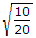= 10.6 μV.

QUESTION: 6

In the television system in India, the odd and even fields are scanned

Solution:

Each field is scanned 25 times but alternately.

QUESTION: 7

Consider the following

1. A signal f(t) and its Hilbert transform fh(t) have the same energy density spectrum
2. A signal f(t) and its Hilbert transform, fh(t) have the same auto correlation function
3. A signal f(t) and its Hilbert transform are mutually orthogonal
Which of the above are true?
Solution:

All are correct.

QUESTION: 8

Vidicon and plumbicon operate on the principle of

Solution:

Photo conduction uses the fact that resistivity of some materials depends on intensity of light falling on their surface.

QUESTION: 9

The typical squelch circuit

Solution:

To suppress noise when carrier is absent, squelch circuit cuts off audio amplifier.

QUESTION: 10

A TV receiver has a width of 60 cm. The aspect ratio is 4/3. The required EHT supply is about

Solution:

EHT supply is 1 kV per diagonal inch.

Height = 60 x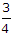= 45 cm.

Diagonal =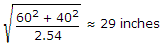.

QUESTION: 11

A narrow band noise n(t) has symmetrical spectrum and has power density spectrum 0.2 x 10-6. The power density of quadrature component is

Solution: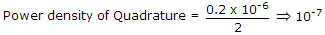.

QUESTION: 12

Solution:

QUESTION: 13

The reactance of coupling capacitors, for DC quantities, is

Solution:

For zero frequency capacitive reactance is infinite.

QUESTION: 14

An AM wave is given by s(t)AM = 20 (1 + 0.5 cos 103t + 0.3 cos 104 t cos 106 t) the modulation index of the envelope is

Solution: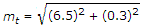.

QUESTION: 15

The modulating frequency in frequency modulation is increased from 10 kHz to 30 kHz. The bandwidth is increased by

Solution:

BW = 2fm(1 + β)

for β >> 1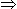B.W. a 2fm .

QUESTION: 16

A sinusoidal signal with peak to peak amplitude of 1.536 volt is quantized into 128 levels using a mid-rise uniform quantizer. The quantization noise power is

Solution: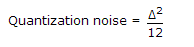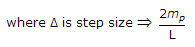.

QUESTION: 17

An FM wave is made to pass through a frequency tripler. If original modulation index is mf, the output of tripler has a modulation index equal to

Solution:

Frequency tripler makes modulation index three times.

QUESTION: 18

A balun provides an impedance transformation of

Solution:

Balun has turn ratio of 2 : 1. Therefore, impedance transformation is 4 : 1.

QUESTION: 19

A signal m(t) with bandwidth 500 Hz is first multiplied by a signal g(t) where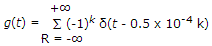. The resulting signal is then passes through an ideal low pass filter with bandwidth 1 kHz the output of the low pass filter would be

Solution:

Multiplication in time domain is equivalent to convolution. According to the property of impulse signal, convolution of a signal m(t) with δ(t) is signal itself.

m(t) x g(t)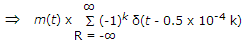m(t).

QUESTION: 20

If γ = (S0/N0)/(Si/Nm) where S0 and S1 are signal power output and signal power input and N0 and Nm are noise power output and noise power input, the value of γ for SSB-SC and DSB-SC amplitude modulated system respectively are

Solution:

SSB - SC and SSB - DB have γ = 1 and are similar from noise point.

QUESTION: 21

Consider the draw backs

2. Serration noise
3. Granular noise
Which of these draw backs does Delta modulation have?
Solution:

In delta modulation the, recovered waveform may be dc whereas the original signal is not dc. This noise is called granular noise.

QUESTION: 22

It is required to increase the maximum range of a radar by a factor of two. The peak power transmitted in the radar system has to be increased by a factor of

Solution:

Range μ (Power)0.25

QUESTION: 23

A source generates three symbols with probabilities 0.25, 0.25, 0.50 at a rate of 3000 symbols per second. Assuming independent generation of symbols, the most efficient source encoder would have average bit rate is as

Solution:

Use Shannon Fano coding.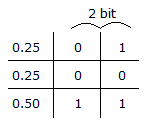(0.25) is encoded as 01Number of bits/sec

= 0.25 x 3000 x 2 + 0.25 x 3000 x 2 + 0.5 x 300045000 bits/sec.

QUESTION: 24

Armstrong F.M. transmitter performs frequency multiplication in stages

Solution: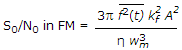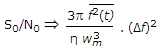where (Δf) is the frequency deviation.

QUESTION: 25

The filter circuit for feeding tweeter has

Solution:

Series capacitance and shunt inductance is high pass circuit.